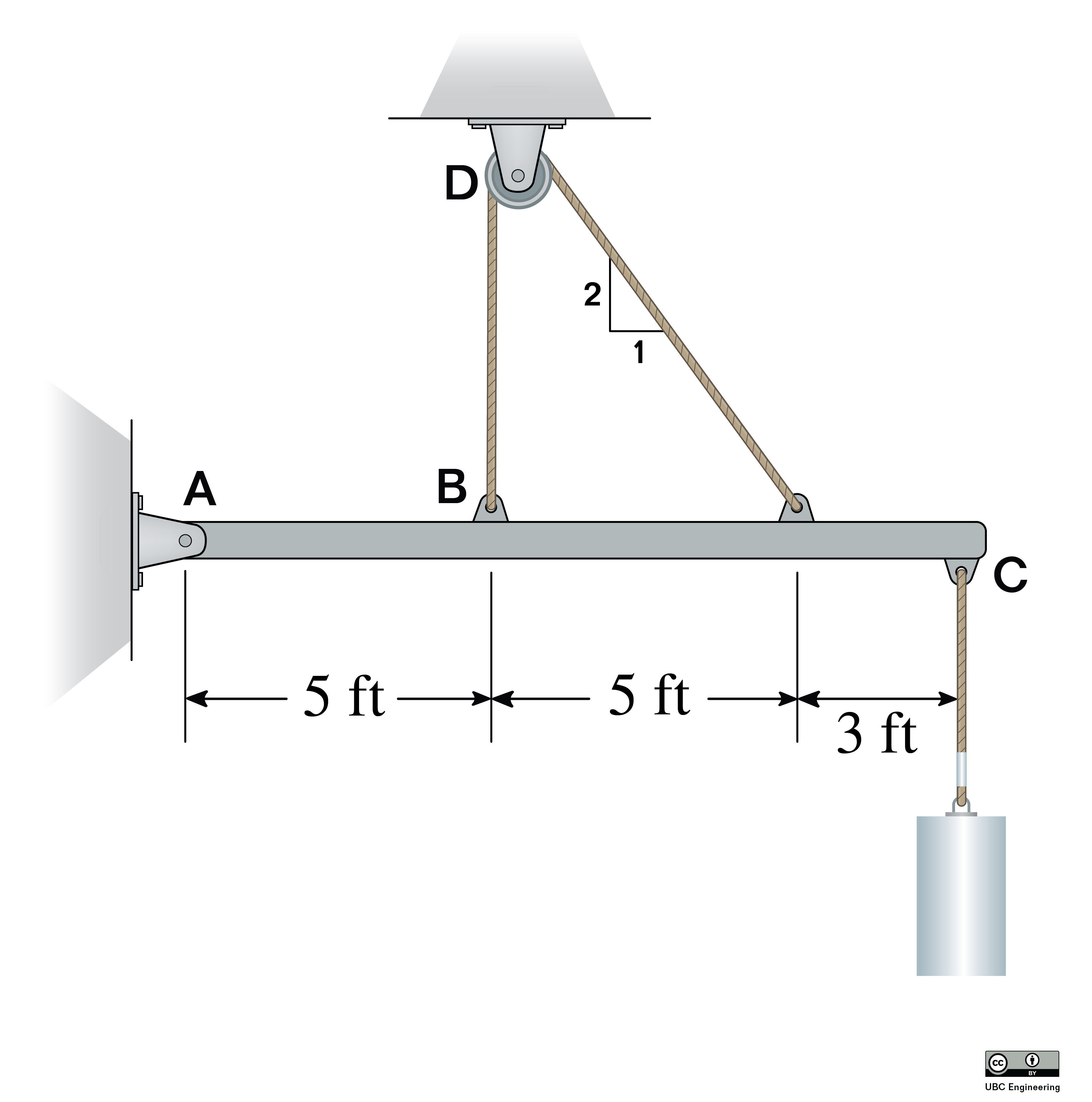# Equilibrium Analysis for a Rigid Body

For an rigid body in static equilibrium, that is a non-deformable body where forces are not concurrent, the sum of both the forces and the moments acting on the body must be equal to zero. The addition of moments (as opposed to particles where we only looked at the forces) adds another set of possible equilibrium equations, allowing us to solve for more unknowns as compared to particle problems.

Moments, like forces, are vectors. This means that our vector equation needs to be broken down into scalar components before we can solve the equilibrium equations. In a two dimensional problem, the body can only have clockwise or counter clockwise rotation (corresponding to rotations about the z axis). This means that a rigid body in a two dimensional problem has three possible equilibrium equations; that is, the sum of force components in the x and y directions, and the moments about the z axis. The sum of each of these will be equal to zero.

For a two dimensional problem, we break our one vector force equation into two scalar component equations.

 $\sum \vec{F}=0$ $\sum F_x=0$ $\sum F_y=0$

The one moment vector equation becomes a single moment scalar equation.

 $\sum \vec{M}=0$ $\sum M_z=0$

If we look at a three dimensional problem we will increase the number of possible equilibrium equations to six. There are three equilibrium equations for force, where the sum of the components in the x, y, and z direction must be equal to zero. The body may also have moments about each of the three axes. The second set of three equilibrium equations states that the sum of the moment components about the x, y, and z axes must also be equal to zero.

We break the forces into three component equations

 $\sum \vec{F}=0$ $\sum F_x=0$ $\sum F_y=0$ $\sum F_z=0$

We break the moments into three component equations

 $\sum \vec{M}=0$ $\sum M_x=0$ $\sum M_y=0$ $\sum M_z=0$

## Finding the Equilibrium Equations:

As with particles, the first step in finding the equilibrium equations is to draw a free body diagram of the body being analyzed. This diagram should show all the force vectors acting on the body. In the free body diagram, provide values for any of the known magnitudes, directions, and points of application for the force vectors and provide variable names for any unknowns (either magnitudes, directions, or distances).

Next you will need to choose the x, y, z axes. These axes do need to be perpendicular to one another, but they do not necessarily have to be horizontal or vertical. If you choose coordinate axes that line up with some of your force vectors you will simplify later analysis.

Once you have chosen axes, you need to break down all of the force vectors into components along the x, y and z directions (see the vectors page in Appendix 1 page for more details on this process). Your first equation will be the sum of the magnitudes of the components in the x direction being equal to zero, the second equation will be the sum of the magnitudes of the components in the y direction being equal to zero, and the third (if you have a 3D problem) will be the sum of the magnitudes in the z direction being equal to zero.

Next you will need to come up with the the moment equations. To do this you will need to choose a point to take the moments about. Any point should work, but it is usually advantageous to choose a point that will decrease the number of unknowns in the equation. Remember that any force vector that travels through a given point will exert no moment about that point. To write out the moment equations simply sum the moments exerted by each force (adding in pure moments shown in the diagram) about the given point and the given axis (x, y, or z) and set that sum equal to zero. All moments will be about the z axis for two dimensional problems, though moments can be about x, y and z axes for three dimensional problems.

Once you have your equilibrium equations, you can solve these formulas for unknowns. The number of unknowns that you will be able to solve for will again be the number or equations that you have.

## Worked Problems:

### Question 1:

The car below has a mass of 1500 lbs with the center of mass 4 ft behind the front wheels of the car. What are the normal forces on the front and the back wheels of the car?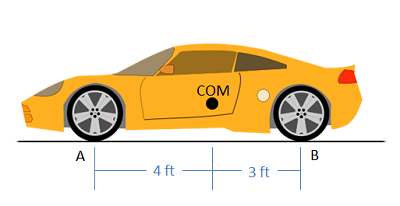Adapted from the public domain image by Ebaychatter0

### Question 2:

A 5 meter long beam has a fixed connection to a wall at point A and a force acting as shown at point B. What are the reaction forces acting on the beam at point A?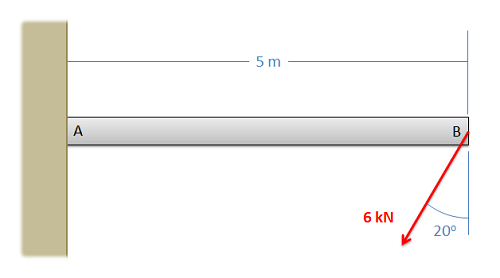### Question 3:

A ladder with negligible mass is supporting 120 lb person as shown below. If the contact point at A is frictionless, and the contact point at B is a rough connection, determine the forces acting at contact points A and B.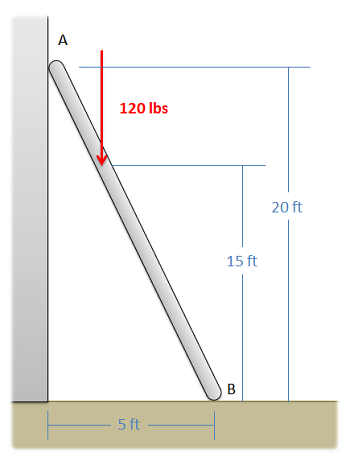### Question 4:

Member ABC is 6 meters long with point B being in the middle. Determine all forces acting on member ABC.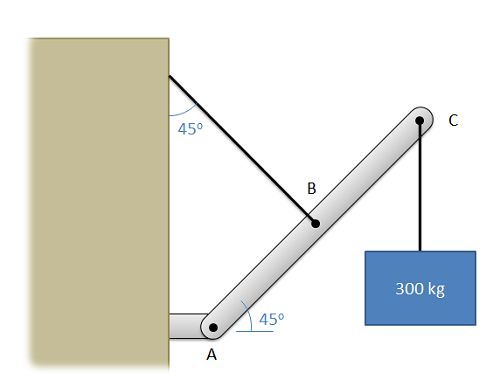### Question 5:

While sitting in a chair, a person exerts the forces in the diagram below. Determine all forces acting on the chair at points A and B. (Assume A is frictionless and B is a rough surface).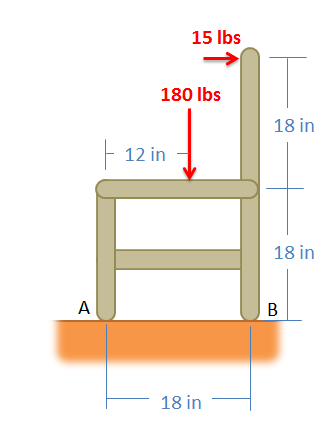### Question 6:

The trailer shown below consists of a deck with a weight of 250 lbs on an axle with wheels with a weight of 350 lbs. Assume the weight forces act in the center of each component. If we wish the tongue weight (Ft) of the unloaded trailer to be 50 lbs, how far from the front must we place the axle(d)?### Question 7:

A 12 in by 24 in flat steel sign is supported by two cables, each 6 inches from the edge of the sign. The sign has a weight of 10 lbs, and the wind is causing the sign to sit at an angle of 10 degrees from vertical (the y axis). If we treat the wind as a point force acting in the negative z direction on the center of the sign, how strong must the wind force be to cause this ten degree angle?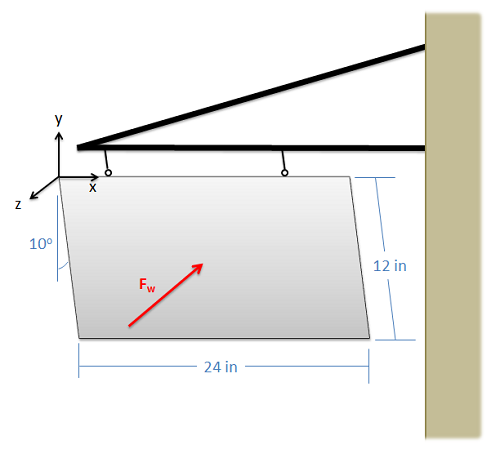### Question 8:

A sixty kilogram acoustic panel is suspended by three cables as shown below. Assuming the panel has a uniformly distributed weight, what is the tension in each of the cables?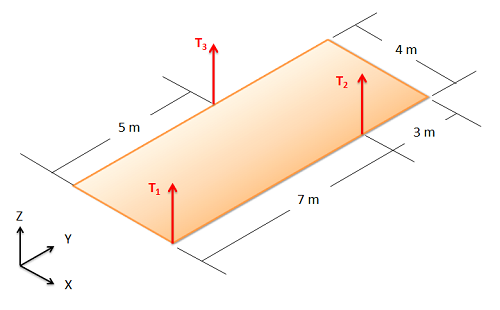### Question 9:

Beam ABC is supporting an 80 pound load at C, while being supported by a pin joint at A and a cable as shown below. Assume that the mass of the beam itself is negligible and that the pulley at D is frictionless. What is the tension in the cable? What are the horizontal and vertical components of the reaction at pin A?